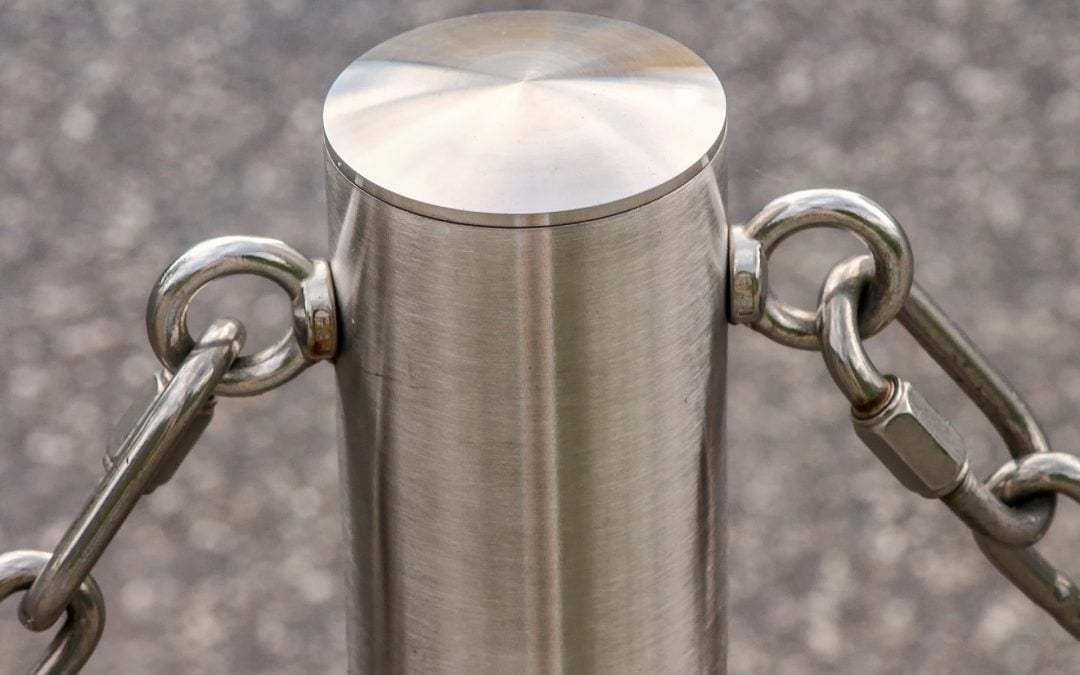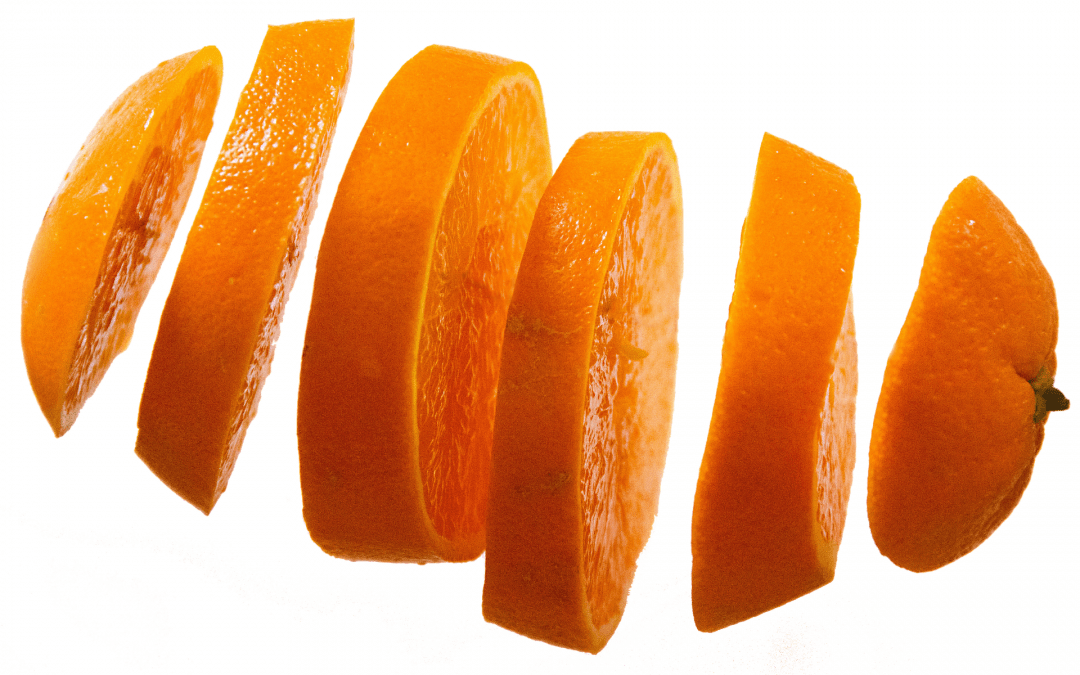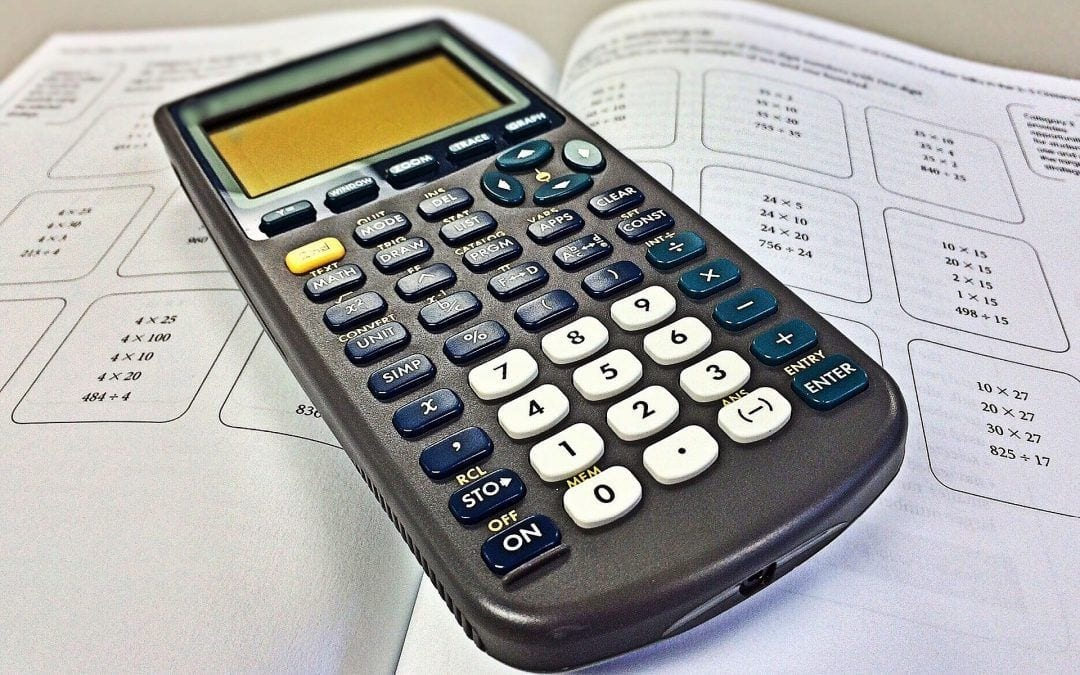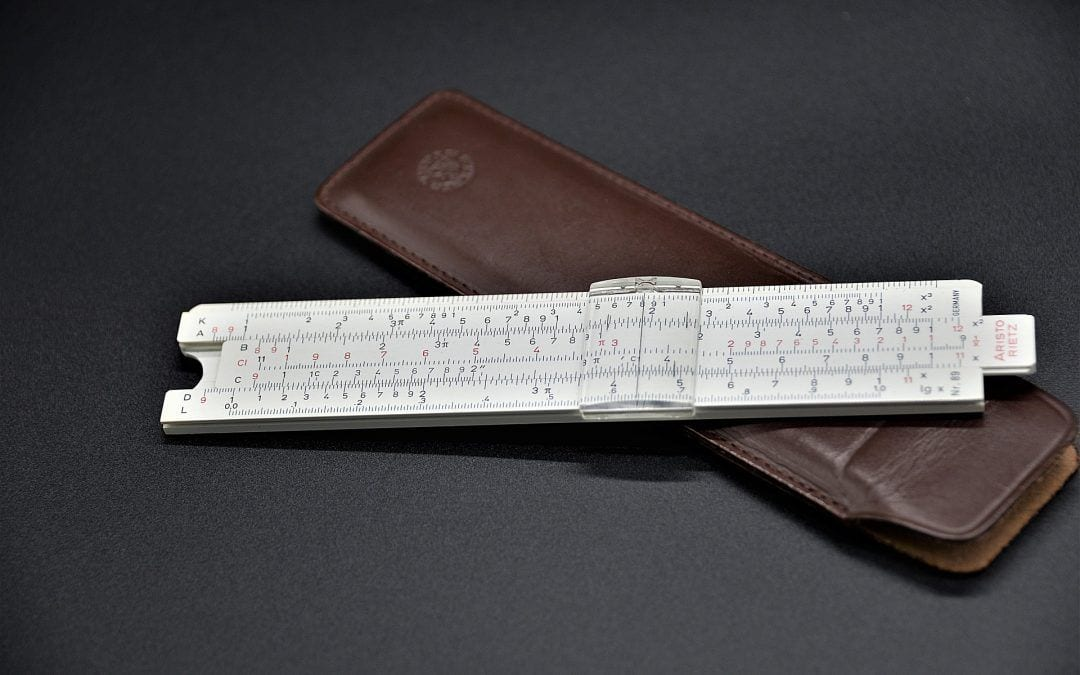## How to use the UPPER formula in Google Sheets

Numbers are what comes to our mind when we think of spreadsheets applications. That is no surprise because they simplify a lot of calculations for us. Thankfully, their support is beyond just the numbers. We also have solid help in the form of many...## How to use the SEARCH formula in Google Sheets

Spreadsheets applications are fantastic utilities at our disposal that not only allows us to tinker with numbers but also with text strings. So along the way, we may find ourselves needing to obtain the position of a substring within a string. We can do that with the...## How to use the CONCAT formula in Google Sheets

While working with strings on spreadsheets, we may have to do many operations such as trimming, extracting, and converting. What about joining two strings together? Sure, we can do that as well. The CONCAT formula in Google Sheets helps us to combine (or concatenate)...## How to use the SPLIT formula in Google Sheets

Spreadsheets have become the first stop for most of us to handle data. And the nature of the data supported by these applications is not limited to numbers. They seem to provide us with all the requisite tools to tackle even the text-based data. In this article, we’ll...## How to use the MID formula in Google Sheets

We use spreadsheets not only for number crunching but also for operating on the text-based data as well. There is a built-in support system to easily convert, trim and combine the strings. We know that we have formulas like LEFT and RIGHT that help us extract the...## How to use the ROWS formula in Google Sheets

Assume that we have a range of cells in a spreadsheet that we are interested in. And we want to know how many rows it has spread across. We could probably do a quick count manually if there are only a few of them. But more often than not, we encounter instances where...## How to use the MMULT formula in Google Sheets

Spreadsheets have come a long way since they were first available, thereby making our lives a lot easier. Today they provide us with a myriad of tools and formulas, which will help us with most of our mathematical and statistical calculations. Therefore, it is no...## How to use the LEFT formula in Google Sheets

The spreadsheets applications of today are extremely versatile and useful tools. While they are known primarily for handling numbers, they also provide multiple utilities that help us operate on text values. In this post, we will be learning about the LEFT formula in...## How to use the LEN formula in Google Sheets

Using spreadsheets we not only can deal with numbers but also with text. We have a multitude of text formulas at our disposal that can help us manipulate the strings. The LEN formula in Google Sheets is one of them that we use very commonly. It simply returns the...## How to use the LOWER formula in Google Sheets

We know we use the spreadsheets applications generally to working with numbers. But we also have a good help in terms of a number of text-based formulas at our disposal. By making use of these, we can manipulate the strings in many ways according to our needs....## How to use the LN formula in Google Sheets

Spreadsheets of today are comprehensive tools that help us with everyday calculations. Be it elementary or advanced, they got a bundle of mathematical functions to offer. The LN formula in Google Sheets helps us to calculate the natural logarithm of a value. It...## How to use the RAND formula in Google Sheets

In fields including but not limited statistics, science, gaming, cryptography etc. the use or random numbers is a commonplace. For instance, statisticians may use randomness in a randomized experiment to test their hypotheses. Because, a random number usually follows...